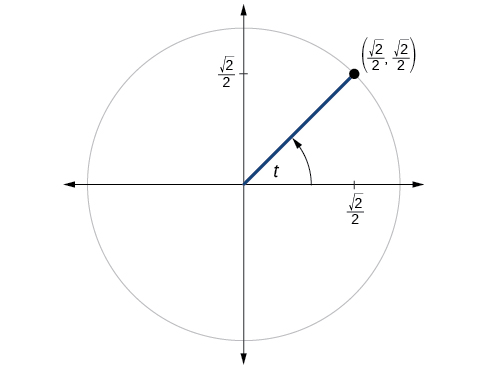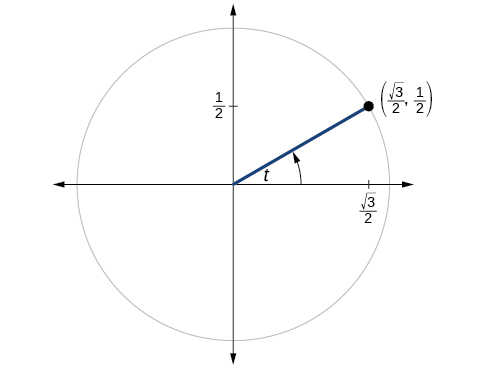7.4 The other trigonometric functions  (Page 7/14)

 Page 7 / 14

If $\mathrm{tan}\text{\hspace{0.17em}}t=\frac{12}{5},$ and $\text{\hspace{0.17em}}0\le t<\frac{\pi }{2},$ find $\text{\hspace{0.17em}}\mathrm{sin}\text{\hspace{0.17em}}t,\mathrm{cos}\text{\hspace{0.17em}}t,\mathrm{sec}\text{\hspace{0.17em}}t,\mathrm{csc}\text{\hspace{0.17em}}t,\text{and}\text{\hspace{0.17em}}\mathrm{cot}\text{\hspace{0.17em}}t.$

If $\text{\hspace{0.17em}}\mathrm{sin}\text{\hspace{0.17em}}t=\frac{\sqrt{3}}{2}\text{\hspace{0.17em}}$ and $\text{\hspace{0.17em}}\mathrm{cos}\text{\hspace{0.17em}}t=\frac{1}{2},$ find $\text{\hspace{0.17em}}\mathrm{sec}\text{\hspace{0.17em}}t,\mathrm{csc}\text{\hspace{0.17em}}t,\mathrm{tan}\text{\hspace{0.17em}}t,$ and $\text{\hspace{0.17em}}\mathrm{cot}\text{\hspace{0.17em}}t.$

$\mathrm{sec}t=2,$ $\mathrm{cot}t=\frac{\sqrt{3}}{3}$

If $\text{\hspace{0.17em}}\mathrm{sin}\text{\hspace{0.17em}}40°\approx 0.643\text{\hspace{0.17em}}$ and $\text{\hspace{0.17em}}\mathrm{cos}\text{\hspace{0.17em}}40°\approx 0.766,$ find $\text{\hspace{0.17em}}\text{sec}\text{\hspace{0.17em}}40°,\text{csc}\text{\hspace{0.17em}}40°,\text{tan}\text{\hspace{0.17em}}40°,$ and $\text{\hspace{0.17em}}\text{cot}\text{\hspace{0.17em}}40°.$

If $\text{\hspace{0.17em}}\text{sin}\text{\hspace{0.17em}}t=\frac{\sqrt{2}}{2},$ what is the $\text{\hspace{0.17em}}\text{sin}\left(-t\right)?$

$-\frac{\sqrt{2}}{2}$

If $\text{\hspace{0.17em}}\text{cos}\text{\hspace{0.17em}}t=\frac{1}{2},$ what is the $\text{\hspace{0.17em}}\text{cos}\left(-t\right)?$

If $\text{\hspace{0.17em}}\text{sec}\text{\hspace{0.17em}}t=3.1,$ what is the $\text{\hspace{0.17em}}\text{sec}\left(-t\right)?$

3.1

If $\text{\hspace{0.17em}}\text{csc}\text{\hspace{0.17em}}t=0.34,$ what is the $\text{\hspace{0.17em}}\text{csc}\left(-t\right)?$

If $\text{\hspace{0.17em}}\text{tan}\text{\hspace{0.17em}}t=-1.4,$ what is the $\text{\hspace{0.17em}}\text{tan}\left(-t\right)?$

1.4

If $\text{\hspace{0.17em}}\text{cot}\text{\hspace{0.17em}}t=9.23,$ what is the $\text{\hspace{0.17em}}\text{cot}\left(-t\right)?$

Graphical

For the following exercises, use the angle in the unit circle to find the value of the each of the six trigonometric functions.$\mathrm{sin}t=\frac{\sqrt{2}}{2},\mathrm{cos}t=\frac{\sqrt{2}}{2},\mathrm{tan}t=1,\mathrm{cot}t=1,\mathrm{sec}t=\sqrt{2},\mathrm{csc}t=\sqrt{2}$$\mathrm{sin}t=-\frac{\sqrt{3}}{2},\mathrm{cos}t=-\frac{1}{2},\mathrm{tan}t=\sqrt{3},\mathrm{cot}t=\frac{\sqrt{3}}{3},\mathrm{sec}t=-2,\mathrm{csc}t=-\frac{2\sqrt{3}}{3}$

Technology

For the following exercises, use a graphing calculator to evaluate to three decimal places.

$\mathrm{csc}\text{\hspace{0.17em}}\frac{5\pi }{9}$

$\mathrm{cot}\text{\hspace{0.17em}}\frac{4\pi }{7}$

–0.228

$\mathrm{sec}\text{\hspace{0.17em}}\frac{\pi }{10}$

$\mathrm{tan}\text{\hspace{0.17em}}\frac{5\pi }{8}$

–2.414

$\mathrm{sec}\text{\hspace{0.17em}}\frac{3\pi }{4}$

$\mathrm{csc}\text{\hspace{0.17em}}\frac{\pi }{4}$

1.414

$\text{tan}\text{\hspace{0.17em}}98°$

$\mathrm{cot}\text{\hspace{0.17em}}33°$

1.540

$\mathrm{cot}\text{\hspace{0.17em}}140°$

$\mathrm{sec}\text{\hspace{0.17em}}310°$

1.556

Extensions

For the following exercises, use identities to evaluate the expression.

If $\text{\hspace{0.17em}}\mathrm{tan}\left(t\right)\approx 2.7,$ and $\text{\hspace{0.17em}}\mathrm{sin}\left(t\right)\approx 0.94,$ find $\text{\hspace{0.17em}}\mathrm{cos}\left(t\right).$

If $\text{\hspace{0.17em}}\mathrm{tan}\left(t\right)\approx 1.3,$ and $\text{\hspace{0.17em}}\mathrm{cos}\left(t\right)\approx 0.61,$ find $\text{\hspace{0.17em}}\mathrm{sin}\left(t\right).$

$\mathrm{sin}\left(t\right)\approx 0.79$

If $\text{\hspace{0.17em}}\mathrm{csc}\left(t\right)\approx 3.2,$ and $\text{\hspace{0.17em}}\mathrm{cos}\left(t\right)\approx 0.95,$ find $\text{\hspace{0.17em}}\mathrm{tan}\left(t\right).$

If $\text{\hspace{0.17em}}\mathrm{cot}\left(t\right)\approx 0.58,$ and $\text{\hspace{0.17em}}\mathrm{cos}\left(t\right)\approx 0.5,$ find $\text{\hspace{0.17em}}\mathrm{csc}\left(t\right).$

$\mathrm{csc}t\approx 1.16$

Determine whether the function $\text{\hspace{0.17em}}f\left(x\right)=2\mathrm{sin}x\text{\hspace{0.17em}}\mathrm{cos}\text{\hspace{0.17em}}x\text{\hspace{0.17em}}$ is even, odd, or neither.

Determine whether the function $\text{\hspace{0.17em}}f\left(x\right)=3{\mathrm{sin}}^{2}x\text{\hspace{0.17em}}\mathrm{cos}\text{\hspace{0.17em}}x+\mathrm{sec}\text{\hspace{0.17em}}x\text{\hspace{0.17em}}$ is even, odd, or neither.

even

Determine whether the function $\text{\hspace{0.17em}}f\left(x\right)=\mathrm{sin}\text{\hspace{0.17em}}x-2{\mathrm{cos}}^{2}x\text{\hspace{0.17em}}$ is even, odd, or neither.

Determine whether the function $\text{\hspace{0.17em}}f\left(x\right)={\mathrm{csc}}^{2}x+\mathrm{sec}\text{\hspace{0.17em}}x\text{\hspace{0.17em}}$ is even, odd, or neither.

even

For the following exercises, use identities to simplify the expression.

$\mathrm{csc}\text{\hspace{0.17em}}t\text{\hspace{0.17em}}\mathrm{tan}\text{\hspace{0.17em}}t$

$\frac{\mathrm{sec}\text{\hspace{0.17em}}t}{\mathrm{csc}\text{\hspace{0.17em}}t}$

$\frac{\mathrm{sin}\text{\hspace{0.17em}}t}{\mathrm{cos}\text{\hspace{0.17em}}t}=\mathrm{tan}\text{\hspace{0.17em}}t$

Real-world applications

The amount of sunlight in a certain city can be modeled by the function $\text{\hspace{0.17em}}h=15\mathrm{cos}\left(\frac{1}{600}d\right),$ where $\text{\hspace{0.17em}}h\text{\hspace{0.17em}}$ represents the hours of sunlight, and $\text{\hspace{0.17em}}d\text{\hspace{0.17em}}$ is the day of the year. Use the equation to find how many hours of sunlight there are on February 10, the 42 nd day of the year. State the period of the function.

The amount of sunlight in a certain city can be modeled by the function $\text{\hspace{0.17em}}h=16\mathrm{cos}\left(\frac{1}{500}d\right),$ where $\text{\hspace{0.17em}}h\text{\hspace{0.17em}}$ represents the hours of sunlight, and $\text{\hspace{0.17em}}d\text{\hspace{0.17em}}$ is the day of the year. Use the equation to find how many hours of sunlight there are on September 24, the 267th day of the year. State the period of the function.

13.77 hours, period: $\text{\hspace{0.17em}}1000\pi$

The equation $\text{\hspace{0.17em}}P=20\mathrm{sin}\left(2\pi t\right)+100\text{\hspace{0.17em}}$ models the blood pressure, $\text{\hspace{0.17em}}P,$ where $\text{\hspace{0.17em}}t\text{\hspace{0.17em}}$ represents time in seconds. (a) Find the blood pressure after 15 seconds. (b) What are the maximum and minimum blood pressures?

The height of a piston, $\text{\hspace{0.17em}}h,$ in inches, can be modeled by the equation $\text{\hspace{0.17em}}y=2\mathrm{cos}\text{\hspace{0.17em}}x+6,$ where $\text{\hspace{0.17em}}x\text{\hspace{0.17em}}$ represents the crank angle. Find the height of the piston when the crank angle is $\text{\hspace{0.17em}}55°.$

7.73 inches

The height of a piston, $\text{\hspace{0.17em}}h,$ in inches, can be modeled by the equation $\text{\hspace{0.17em}}y=2\mathrm{cos}\text{\hspace{0.17em}}x+5,$ where $\text{\hspace{0.17em}}x\text{\hspace{0.17em}}$ represents the crank angle. Find the height of the piston when the crank angle is $\text{\hspace{0.17em}}55°.$

Angles

For the following exercises, convert the angle measures to degrees.

$45°$

$-\frac{5\pi }{3}$

For the following exercises, convert the angle measures to radians.

$-210°$

$-\frac{7\pi }{6}$

$180°$

Find the length of an arc in a circle of radius 7 meters subtended by the central angle of $\text{\hspace{0.17em}}85°.$

10.385 meters

A laser rangefinder is locked on a comet approaching Earth. The distance g(x), in kilometers, of the comet after x days, for x in the interval 0 to 30 days, is given by g(x)=250,000csc(π30x). Graph g(x) on the interval [0, 35]. Evaluate g(5)  and interpret the information. What is the minimum distance between the comet and Earth? When does this occur? To which constant in the equation does this correspond? Find and discuss the meaning of any vertical asymptotes.
The sequence is {1,-1,1-1.....} has
how can we solve this problem
Sin(A+B) = sinBcosA+cosBsinA
Prove it
Eseka
Eseka
hi
Joel
June needs 45 gallons of punch. 2 different coolers. Bigger cooler is 5 times as large as smaller cooler. How many gallons in each cooler?
7.5 and 37.5
Nando
find the sum of 28th term of the AP 3+10+17+---------
I think you should say "28 terms" instead of "28th term"
Vedant
the 28th term is 175
Nando
192
Kenneth
if sequence sn is a such that sn>0 for all n and lim sn=0than prove that lim (s1 s2............ sn) ke hole power n =n
write down the polynomial function with root 1/3,2,-3 with solution
if A and B are subspaces of V prove that (A+B)/B=A/(A-B)
write down the value of each of the following in surd form a)cos(-65°) b)sin(-180°)c)tan(225°)d)tan(135°)
Prove that (sinA/1-cosA - 1-cosA/sinA) (cosA/1-sinA - 1-sinA/cosA) = 4
what is the answer to dividing negative index
In a triangle ABC prove that. (b+c)cosA+(c+a)cosB+(a+b)cisC=a+b+c.
give me the waec 2019 questionsByByByBy Rhodes